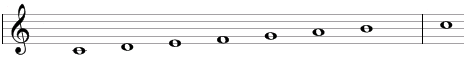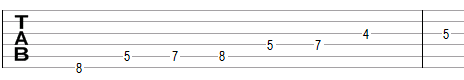# Ionian scale

The Ionian scale is a primary heptatonic scale that can be built using the following steps between notes: 1, 1, ½, 1, 1, 1, ½.

One example of this scale is C, D, E, F, G, A, B. This example is shown below in traditional notation and in guitar tablature notation.The Ionian scale the first mode of standard major scale (the major scale itself) or the third mode of the natural minor scale.

The Ionian scale is a "primary" heptatonic scale (heptonia prima) as the intervals between any two adjacent notes are equal either to one or to two semitones (1 step or ½ steps) and as the two single-semitone intervals (between B and C and between E and F in the example above) are spaced as evenly as possible. Going up from the single-semitone interval between B and C we will find two two-semitone intervals - between C and D and D and E – before we get to the second single semitone interval between E and F. Going down from the single-semitone interval between B and C we will encounter three two-semitone intervals – between A and B, G and A, and F and G – before we reach again the single-semitone interval between E and F.

## Modes of the Ionian scale

The following are modes of the Ionian scale.

1. Ionian, or major, with steps 1, 1, ½, 1, 1, 1, ½ (C, D, E, F, G, A, B in the example above).
2. Dorian, with steps 1, ½, 1, 1, 1, ½, 1 (D, E, F, G, A, B, C).
3. Phrygian, with steps ½, 1, 1, 1, ½, 1, 1 (E, F, G, A, B, C, D).
4. Lydian, with steps 1, 1, 1, ½, 1, 1, ½ (F, G, A, B, C, D, E).
5. Mixolydian, with steps 1, 1, ½, 1, 1, ½, 1 (G, A, B, C, D, E, F).
6. Aeolian, with steps 1, ½, 1, 1, ½, 1, 1 (A, B, C, D, E, F, G).
7. Locrian, with steps ½, 1, 1, ½, 1, 1, 1 (B, C, D, E, F, G, A).

## Three-note chords on the Inonian scale

The following are common triads built on the notes of the Ionian scale.

• On the root of the scale (on the tonic): major chord (e.g., C composed of C, E, G) or suspended chords (Csus4 = C, F, G and Csus2 = C, D, G).
• On the second note (on the supertonic): minor chord (Dm = D, F, A) or suspended chords (Dsus4 = D, G, A and Dsus2 = D, E, A).
• On the third note (on the mediant): minor chord (Em = E, G, B) or suspended chord (Esus4 = E, A, B).
• On the fourth note (on the subdominant): major chord (F = F, A, C) or suspended chord (Fsus2 = F, G, C).
• On the fifth note (on the dominant): major chord (G = G, B, D) or suspended chords (Gsus4 = G, C, D and Gsus2 = G, A, D).
• On the sixth note (on the submediant): minor chord (Am = A, C, E) or suspended chords (Asus4 = A, D, E and Asus2 = A, B, E).
• On the seventh note (on the leading tone: diminished chord (Bdim = B, D, F).

## Four-note chords on the Ionian scale

The following are seventh chords built on the notes of the Ionian scale.

• On the first note: major seventh chord (e.g., Cmaj7 composed of C, E, G, B).
• On the second note: half-diminished seventh chord (minor seventh chord with a flat fifth, Bm7b5 = B, D, F, A).
• On the third note: minor seventh chord (Emin7 = E, G, B, D).
• On the fourth note: major seventh chord (Fmaj7 = F, A, C, E).
• On the fifth note: dominant seventh chord (G7 = G, B, D, F).
• On the sixth note: minor seventh chord (Amin7 = A, C, E, G).
• On the seventh note: minor seventh chord (Dmin7 = D, F, A, C).

## Intervals on the Ionian scale

The Ionian scale is composed of the following intervals.

• A major second, e.g., the interval between C and D is equal to two semitones.
• A major third, e.g., the interval between C and E is equal to four semitones.
• A perfect fourth, e.g., the interval between C and F is equal to five semitones.
• A perfect fifth, e.g., the interval between C and G is equal to seven semitones.
• A major sixth, e.g., the interval between C and A is equal to nine semitones.
• A major seventh, e.g., the interval between C and B is equal to eleven semitones.

Scale, Scale (index)

### Filtered HTML

• Freelinking helps you easily create HTML links. Links take the form of [[indicator:target|Title]]. By default (no indicator): Click to view a local node.